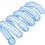# Deriving Newton's 1st Law for Three-Dimensional Motion

Here is a derivation of Newton's 1st law of motion in three-dimensions. The derivation is based on the classical least-action principle. It should be evident by the end that the same process works for any number of dimensions.

Suppose a particle goes from $(x_1,y_1,z_1)$ to $(x_2,y_2,z_2)$ between time $t_1$ and time $t_2$. Suppose also that space is potential-free (and thus force-free). The action is defined as (where $E$ is the kinetic energy and $U$ is the potential energy):

$S = \int_{t_1}^{t_2} ( E - U) \, dt = \int_{t_1}^{t_2} E \, dt$

The particle will follow the path between the two points which minimizes the action. Suppose we discretize the path into $N$ constant-velocity periods. $N$ can be made arbitrarily large. For each period, the velocity is $v_k$. The action becomes:

$S = \frac{m}{2} \, \Sigma_{k=1}^{k=N} (v_{xk} ^2 + v_{yk} ^2 + v_{zk} ^2) \, \Delta t \\ \Delta t = \frac{t_2 - t_1}{N}$

The actual path must minimize the action while satisfying the constraints corresponding to the changes in position. We can use the method of Lagrange multipliers with the following Lagrangian:

$L = \frac{m}{2} \, \Sigma_{k=1}^{k=N} (v_{xk} ^2 + v_{yk} ^2 + v_{zk} ^2) \, \Delta t \\ + \lambda_x \Big ((\Sigma_{k=1}^{k=N} v_{xk} \, \Delta t) - (x_2 - x_1) \Big) \\ + \lambda_y \Big ((\Sigma_{k=1}^{k=N} v_{yk} \, \Delta t) - (y_2 - y_1) \Big) \\ + \lambda_z \Big ((\Sigma_{k=1}^{k=N} v_{zk} \, \Delta t) - (z_2 - z_1) \Big)$

Taking partial derivatives with respect to the period-specific velocities and setting to zero (per standard practice) results in:

$\frac{\partial{L}}{\partial{v_{x1}}} = m \, v_{x1} \, \Delta t + \lambda_x \, \Delta t = 0 \implies v_{x1} = -\frac{\lambda_x}{m} \\ \frac{\partial{L}}{\partial{v_{y1}}} = m \, v_{y1} \, \Delta t + \lambda_y \, \Delta t = 0 \implies v_{y1} = -\frac{\lambda_y}{m} \\ \frac{\partial{L}}{\partial{v_{z1}}} = m \, v_{z1} \, \Delta t + \lambda_z \, \Delta t = 0 \implies v_{z1} = -\frac{\lambda_z}{m} \\ \frac{\partial{L}}{\partial{v_{x2}}} = m \, v_{x2} \, \Delta t + \lambda_x \, \Delta t = 0 \implies v_{x2} = -\frac{\lambda_x}{m} \\ \frac{\partial{L}}{\partial{v_{y2}}} = m \, v_{y2} \, \Delta t + \lambda_y \, \Delta t = 0 \implies v_{y2} = -\frac{\lambda_y}{m} \\ \frac{\partial{L}}{\partial{v_{z2}}} = m \, v_{z2} \, \Delta t + \lambda_z \, \Delta t = 0 \implies v_{z2} = -\frac{\lambda_z}{m} \\ etc. \\ \implies v_{x1} = v_{x2} = v_{xk} \\ \implies v_{y1} = v_{y2} = v_{yk} \\ \implies v_{z1} = v_{z2} = v_{zk}$

Thus, for motion in potential-free (force-free) three-dimensional space, the least-action principle dictates that the vector velocity must be constant.Note by Steven Chase
1 year, 4 months ago

This discussion board is a place to discuss our Daily Challenges and the math and science related to those challenges. Explanations are more than just a solution — they should explain the steps and thinking strategies that you used to obtain the solution. Comments should further the discussion of math and science.

When posting on Brilliant:

• Use the emojis to react to an explanation, whether you're congratulating a job well done , or just really confused .
• Ask specific questions about the challenge or the steps in somebody's explanation. Well-posed questions can add a lot to the discussion, but posting "I don't understand!" doesn't help anyone.
• Try to contribute something new to the discussion, whether it is an extension, generalization or other idea related to the challenge.

MarkdownAppears as
*italics* or _italics_ italics
**bold** or __bold__ bold
- bulleted- list
• bulleted
• list
1. numbered2. list
1. numbered
2. list
Note: you must add a full line of space before and after lists for them to show up correctly
paragraph 1paragraph 2

paragraph 1

paragraph 2

[example link](https://brilliant.org)example link
> This is a quote
This is a quote
    # I indented these lines
# 4 spaces, and now they show
# up as a code block.

print "hello world"
# I indented these lines
# 4 spaces, and now they show
# up as a code block.

print "hello world"
MathAppears as
Remember to wrap math in $$ ... $$ or $ ... $ to ensure proper formatting.
2 \times 3 $2 \times 3$
2^{34} $2^{34}$
a_{i-1} $a_{i-1}$
\frac{2}{3} $\frac{2}{3}$
\sqrt{2} $\sqrt{2}$
\sum_{i=1}^3 $\sum_{i=1}^3$
\sin \theta $\sin \theta$
\boxed{123} $\boxed{123}$

Sort by:

Just solved the problem. Thanks for posting it. It was a nice one.

- 1 year, 4 months ago

Insightful analysis.

On a slightly unrelated yet interesting note, I suggest you look up Fermat's principle of least time. The principle goes on to say that the actual path traversed by a 'ray' of light is that which can be traversed in the shortest duration of time. Using this method, the laws of reflection and refraction of light can be derived. This is another variant of the Least Action Principle.

- 1 year, 4 months ago

The problem is up now in the Calculus section

- 1 year, 4 months ago

I am going to post a problem on the "least time" principle soon. Should be fun

- 1 year, 4 months ago